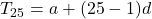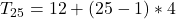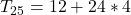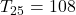7 th term and 14 th term of an Arithmetic sequence are 36 and 64 respectively , find the common difference and ,25th term

Question

7 th term and 14 th term of an Arithmetic sequence are 36 and 64 respectively , find the common difference and ,25th term

in progress 0
5 months 2021-08-28T08:45:26+00:00 1 Answers 0 views 0

(a) The common difference is 4

(b) The 25th term is 108

Step-by-step explanation:

Given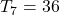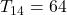Solving (a): The common difference

The nth term of an AP is: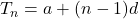For the 7th term, we have: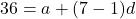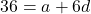For the 14th term, we have: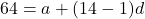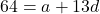Subtract both equations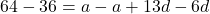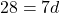Divide by 7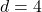Solving (b): The 25th term

First, we calculate the first term (a)

The 7th term of the progression is:Substitute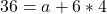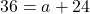Subtract 24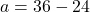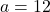The 25th term is: